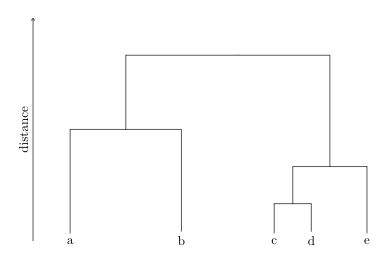{{ message }}

Instantly share code, notes, and snippets.

# dahoo/dendrogram.tex

Last active Aug 28, 2022
Hierarchical Clustering Dendrogram in Latex
This file contains bidirectional Unicode text that may be interpreted or compiled differently than what appears below. To review, open the file in an editor that reveals hidden Unicode characters. Learn more about bidirectional Unicode characters
 \begin{tikzpicture}[sloped] \node (a) at (-6,0) {a}; \node (b) at (-3,0) {b}; \node (c) at (-0.5,0) {c}; \node (d) at (0.5,0) {d}; \node (e) at (2,0) {e}; \node (ab) at (-4.5,3) {}; \node (cd) at (0,1) {}; \node (cde) at (1,2) {}; \node (all) at (-1.5,5) {}; \draw (a) |- (ab.center); \draw (b) |- (ab.center); \draw (c) |- (cd.center); \draw (d) |- (cd.center); \draw (e) |- (cde.center); \draw (cd.center) |- (cde.center); \draw (ab.center) |- (all.center); \draw (cde.center) |- (all.center); \draw[->,-triangle 60] (-7,0) -- node[above]{distance} (-7,6); \end{tikzpicture}

### sunandabansal commented Jul 17, 2021

Thank you for sharing this gist. I was getting an error because of -triangle 60 in line 21, so I removed that part -

\begin{tikzpicture}[sloped]
\node (a) at (-6,0) {a};
\node (b) at (-3,0) {b};
\node (c) at (-0.5,0) {c};
\node (d) at (0.5,0) {d};
\node (e) at (2,0) {e};
\node (ab) at (-4.5,3) {};
\node (cd) at (0,1) {};
\node (cde) at (1,2) {};
\node (all) at (-1.5,5) {};

\draw  (a) |- (ab.center);
\draw  (b) |- (ab.center);
\draw  (c) |- (cd.center);
\draw  (d) |- (cd.center);
\draw  (e) |- (cde.center);
\draw  (cd.center) |- (cde.center);
\draw  (ab.center) |- (all.center);
\draw  (cde.center) |- (all.center);

\draw[->] (-7,0) -- node[above]{distance} (-7,6);
\end{tikzpicture}

And the result was (for reference) -### sunandabansal commented Jul 18, 2021

A little enhance version of the above script -

\begin{tikzpicture}[sloped]
\node (a) at (-6,0) {a};
\node (b) at (-3,0) {b};
\node (c) at (-0.5,0) {c};
\node (d) at (0.5,0) {d};
\node (e) at (2,0) {e};
\node (ab) at (-4.5,3) {};
\node (cd) at (0,1) {};
\node (cde) at (1,2) {};
\node (all) at (-1.5,4.5) {};

\draw  (a) |- (ab.center);
\draw  (b) |- (ab.center);
\draw  (c) |- (cd.center);
\draw  (d) |- (cd.center);
\draw  (e) |- (cde.center);
\draw  (cd.center) |- (cde.center);
\draw  (ab.center) |- (all.center);
\draw  (cde.center) |- (all.center);

\draw[<-] (-10,0) -- node[above]{similarity} (-10,5);
\draw[->] (-9,0) -- node[above]{distance} (-9,5);

\draw (-7,0) -- (-7,5);

\foreach \y in {0,1,2,3,4,5}
\draw[shift={(0,\y)},color=black] (-7,0) -- (-7.1,0);

\node[left] at (-7.1,0) {$1.0$} ;
\node[left] at (-7.1,1) {$0.8$} ;
\node[left] at (-7.1,2) {$0.6$} ;
\node[left] at (-7.1,3) {$0.4$} ;
\node[left] at (-7.1,4) {$0.2$} ;
\node[left] at (-7.1,5) {$0.0$} ;

\draw[dashed,color=gray] (-7,3.5) -- node[at end, right]{cut-off} (2.5,3.5);
\draw[color=gray] (-7,3.5) -- (-7.1,3.5) node[left] {$0.3$} ;
\end{tikzpicture}

to join this conversation on GitHub. Already have an account? Sign in to comment